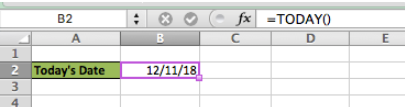Get instant live expert help with Excel or Google Sheets“My Excelchat expert helped me in less than 20 minutes, saving me what would have been 5 hours of work!”

#### Post your problem and you’ll get Expert help in seconds.

Your message must be at least 40 characters
Our professional Expert are available now. Your privacy is guaranteed.

# How to Get a Date to Automatically Update in Excel

It is very simple to automatically generate a new date value each day. By using the TODAY function, Excel users of all levels will be able to automatically reference the current date each day after reviewing this tutorial

Final Formula: `TODAY()`

## Applying the TODAY function

We can automatically generate the current date for each day by using the TODAY function

TODAY Syntax:

`=TODAY()`

• In cell B2, enter this formula =TODAY()Figure 1 – Applying TODAY formula

We can see in this Example, the formula in B2 returns today’s date. Tomorrow, the formula will automatically update to tomorrow’s date. Be sure to set the formula calculation options to automatic

## Instant Connection to an Expert through our Excelchat Service:

Most of the time, the problem you will need to solve will be more complex than a simple application of a formula or function. If you want to save hours of research and frustration, try our live Excelchat service! Our Excel Experts are available 24/7 to answer any Excel question you may have. We guarantee a connection within 30 seconds and a customized solution within 20 minutes.

### Did this post not answer your question? Get a solution from connecting with the expert.Another blog reader asked this question today on Excelchat:
Solution examplesI would need a formula what copies from my DataInput sheet into another one daily the first two column A and B.
Solved by K. S. in 33 minsI would need a formula what copies from my DataInput sheet into another one daily the first two column A and B.
Solved by B. C. in 60 minsI need the formula for time accrual to auto populate in E4 based on certain criteria. example: B4 = hire date E4= accrual rate If todays date is equal to or greater than 60 days but less than 1 year from B4 date then E4 = 1 If todays date is equal to or greater than 3 years but less than 4 years from B4 date then E4 = 1.08 If todays date is equal to or greater than 5 years but less than 9 years from B4 date then E4 = 1.5 If todays date is equal to or greater than 10 years but less than 14 years from B4 date then E4 = 1.58 If todays date is equal to or greater than 15 years from B4 date then E4 = 1.92
Solved by A. H. in 60 minsHello. I would like to create a drop down option to choose Square Feet or Square Meters. Next column they insert the amount and the next it converts the value to whatever is chosen from the drop down option. Can you help please?
Solved by Z. Q. in 39 minsHi there. I need a column to automatically calculate the numbers of days from a start time to today's date.
Solved by T. F. in 16 mins## Subscribe to Excelchat.coAnother blog reader asked this question today on Excelchat: